# Division With Trailing Zeros: Problem Type 1

### Trailing zeros

The zeros at the end of a number are known as trailing zeros. For example, 1200, 70, 3000…have trailing zeros.

In this lesson, we are dealing with problems involving division of numbers with trailing zeros

For example, 1800 ÷ 6 = 300

Divide 1500 ÷ 5

### Solution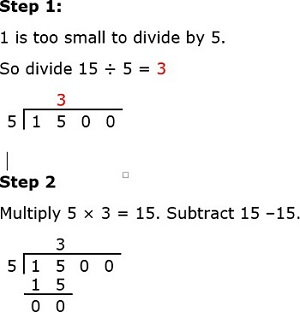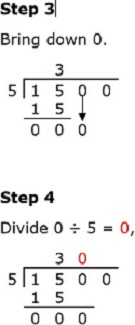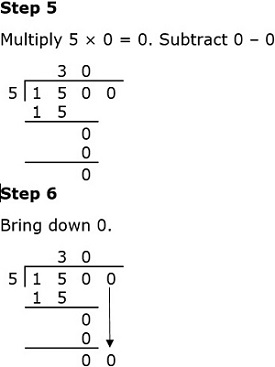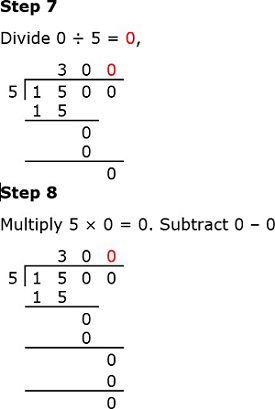So 1500 ÷ 5 = 300

We can check if this is true by multiplying

5 × 300 = 1500. So 300 is the correct quotient.

Divide 400 ÷ 8

### Solution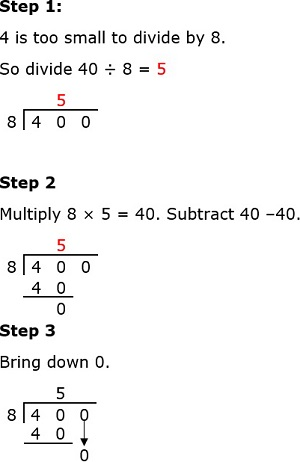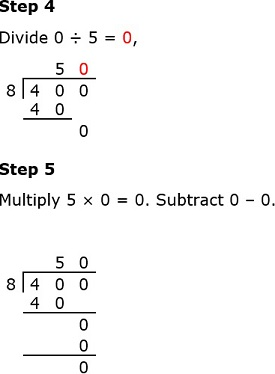So 400 ÷ 8 = 50